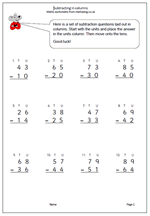# Subtraction in columnsIt is suggested in the new Maths Programme of Study that children in Year 2 should get used to recording addition nand subtraction in columns, both to support place value and to prepare for formal written methods with larger numbers in later years.

Here we have a subtraction worksheet laid out in columns. There is no ‘borrowing’ so it is a simple matter of subtracting the units and subtracting the tens.

Subtract 2-digit numbers in columns

## One thought on “Subtraction in columns”

1.Akhila says:

Column addition and subtraction is the best way to get familiarized with the place values and their significance. Kids need to start practicing these worksheets inorder to take up more challenges in the higher grades.